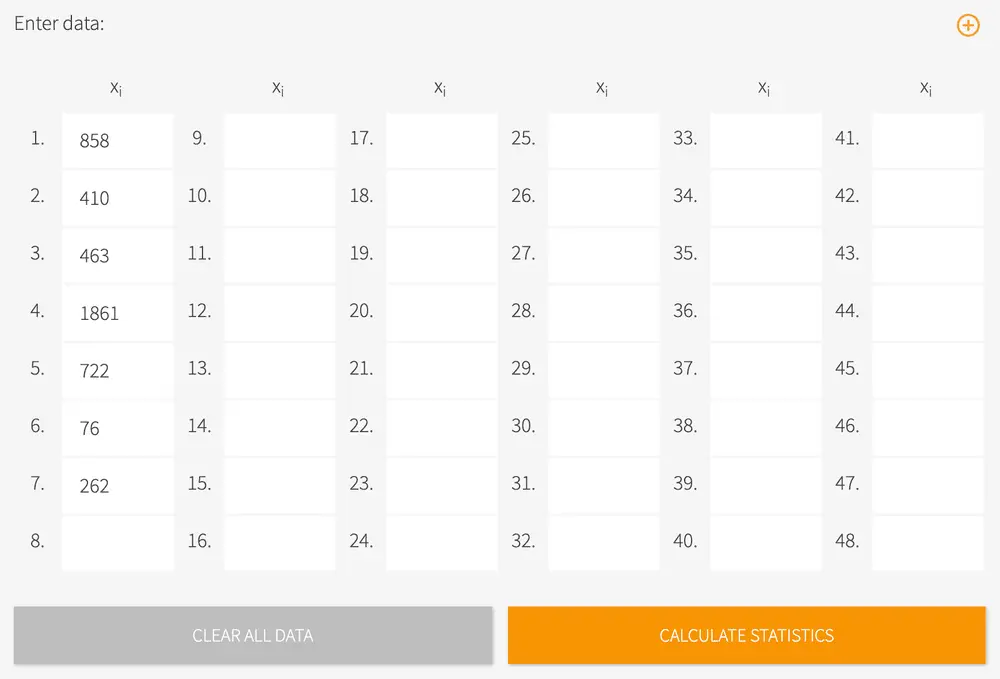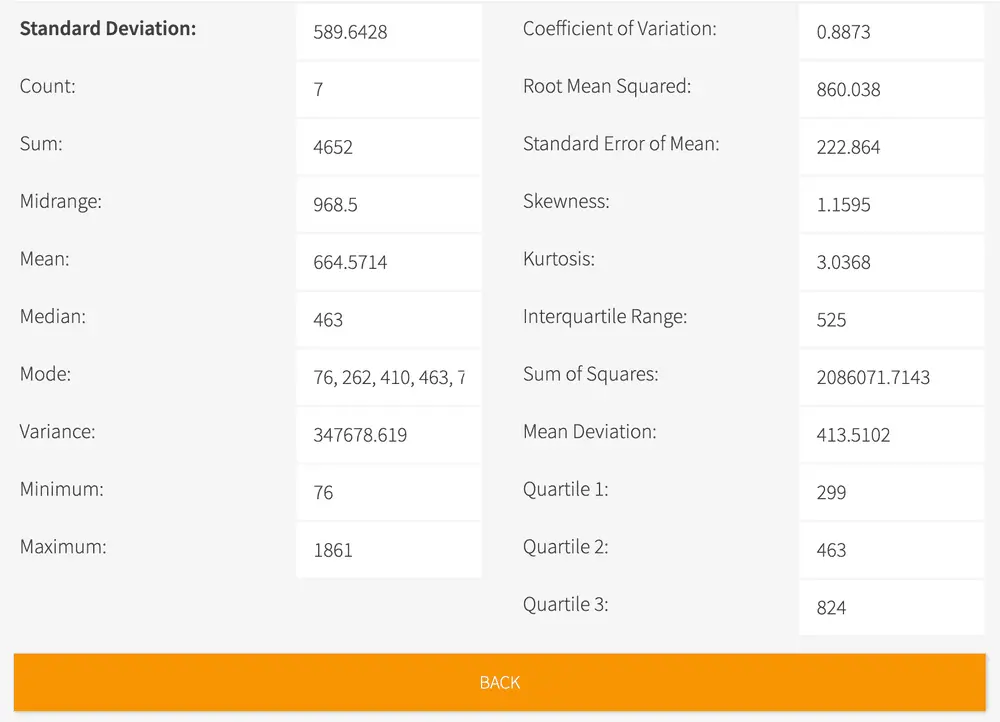# Standard Deviation Calculator

• Standard deviation is a statistical measure of variation or dispersion between values in a data set or in other words, how much the numbers in the data set are likely to differ from the mean value.
• Our standard deviation calculator is the most sophisticated and comprehensive standard deviation calculator online.
• It does much more than just calculating the standard deviation. It also gives you mean, median, variance, coefficient of variance and much more!
• Just click the "start calculator" button below and get started. Our calculator is very easy to use.# Standard Deviation Calculator

#### What is standard deviation?

Standard deviation, also called “the square root of the variance,” is the statistic that measures the dispersion of a dataset relative to its mean. In layman's terms, it is the measure of how spread out numbers are. Standard deviation uses the Greek letter sigma symbol σ.

Variance is the average of the squared differences from the Mean. The square root of the variance can be calculated by finding each data point's deviation relative to the mean.

To calculate standard deviation, determine first the simple average or the mean of the numbers. Next, subtract the mean for each number, square it, and then average the result to get the variance. Lastly, calculate the square root of the variance, and that is your standard deviation.

To help students and professionals solve their math problems conveniently and quickly, Meta-calculator.com provides online calculators that can be accessed for free. You can rely on our online standard deviation calculator not just for solving the standard deviation but also for determining the mean, median, variance, coefficient of variance, and other relevant statistical data.

#### How to use our online standard deviation calculator?

Enter all the data values in the table and click the “Calculate Statistics” button.The online calculator will give the standard deviation result and provide data such as the mean, median, variance, mean deviations, and a lot more.#### Standard deviation calculator handouts & worksheets

Here are a few resources where you can get free handouts and worksheets to test your math skills. Click the link to the desired worksheet to open the PDF file. All files are downloadable for free!